Home / Basic Electrical / Parallel Circuit Definition | Parallel Circuit Examples

# Parallel Circuit Definition | Parallel Circuit Examples

Want create site? Find Free WordPress Themes and plugins.

## Parallel Circuit Definition

Resistors are said to be connected in parallel when the same voltage appears across every component. With different resistance values, different currents flow through each resistor.

The total current taken from the supply is the sum of all the individual resistor currents. The equivalent resistance of a parallel resistor circuit is most easily calculated by using the reciprocal of each individual resistor value.

## Parallel Circuit Characteristics

1. Two resistors connected in parallel may be used as a current divider.
2. In a parallel circuit, as in series circuit, the total power supplied is the sum of the powers dissipated in the individual components.
3. Open-circuit and short-circuit conditions in a parallel circuit have an effect on the supply current.

Parallel Connected Resistors

Resistors are connected in parallel when the circuit has two terminals that are common to every resistor. Figure 1 shows two resistors (R1 and R2) are connected in parallel, with same voltage applied from a power supply. Thus, it can be stated,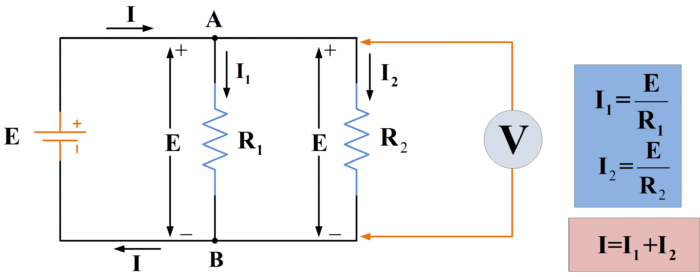Fig.1: Circuit Diagram for Parallel Connected Resistors

Resistors are connected in parallel when the same voltage is applied across each resistor.

## Current Levels

The parallel-resistor circuit diagram in figure 1 shows that different currents flow in each parallel component. As illustrated, the current through each resistor is

\begin{align} & {{I}_{1}}=\frac{E}{{{R}_{1}}} \\ & and \\ & {{I}_{2}}=\frac{E}{{{R}_{2}}} \\\end{align}

Now, look at the current directions in figure 1 with respect to junction A.  I1 flowing through R1 is flowing away from junction A, and I2 flowing through R2 is also flowing away from A. The supply current I is flowing towards A. Also, I, I1, and I2 are the only currents entering or leaving the junction A. Consequently,

$I={{I}_{1}}+{{I}_{2}}$

The same reasoning at junction B, where I1 and I2 are entering and I is leaving B, also gives

$I={{I}_{1}}+{{I}_{2}}$

In the case where there are n resistors in parallel, the supply current is

$\begin{matrix} I={{I}_{1}}+{{I}_{2}}+{{I}_{3}}+\cdots +{{I}_{n}} & \cdots & (1) \\\end{matrix}$

## Kirchhoff’s Current Law

The rule about currents entering and leaving a junction is defined in Kirchhoff’s current law:

KCL DefinitionThe algebraic sum of the currents entering or a point in an electric circuit must equal the algebraic sum of the currents leaving that point.

## Parallel Circuit Example 1

The parallel resistors shown in figure 1 have values of R1=12Ω and R2=15Ω. The supply voltage is E=9V. Calculate the current that flows through each resistor and the total current drawn from the battery.

Solution

\begin{align} & {{I}_{1}}=\frac{E}{{{R}_{1}}}=\frac{9V}{12\Omega }=0.75A \\ & {{I}_{2}}=\frac{E}{{{R}_{2}}}=\frac{9V}{15\Omega }=0.6A \\ & I={{I}_{1}}+{{I}_{2}}=1.35A \\\end{align}

## Parallel Equivalent Resistance

Consider the case of four resistors in parallel, as shown in figure 2.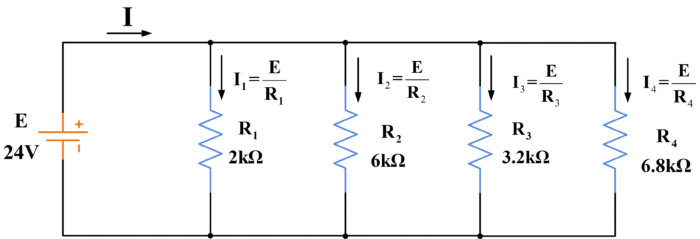Fig.2: Four-Resistor Parallel Circuit

From equation (1), the battery current is

$I={{I}_{1}}+{{I}_{2}}+{{I}_{3}}+{{I}_{4}}$

Which can be rewritten as

\begin{align} & I=\frac{E}{{{R}_{1}}}+\frac{E}{{{R}_{2}}}+\frac{E}{{{R}_{3}}}+\frac{E}{{{R}_{4}}} \\ & or \\ & I=E\left( \frac{1}{{{R}_{1}}}+\frac{1}{{{R}_{2}}}+\frac{1}{{{R}_{3}}}+\frac{1}{{{R}_{4}}} \right) \\\end{align}

For n resistors in parallel, this becomes

$\begin{matrix} I=E\left( \frac{1}{{{R}_{1}}}+\frac{1}{{{R}_{2}}}+\frac{1}{{{R}_{3}}}+\cdots +\frac{1}{{{R}_{n}}} \right) & \cdots & (2) \\\end{matrix}$

If all the resistors in parallel could be replaced by just one resistance that could draw the same current from the battery, the equation for current would be written

\begin{align} & I=\frac{E}{R} \\ & or \\ & I=E(\frac{1}{R}) \\\end{align}

Where

$\begin{matrix} \frac{1}{R}=\frac{1}{{{R}_{1}}}+\frac{1}{{{R}_{2}}}+\frac{1}{{{R}_{3}}}+\cdots +\frac{1}{{{R}_{n}}} & \cdots & (3) \\\end{matrix}$

Thus it is seen that

The reciprocal of the equivalent resistance of several resistors in parallel is equal to the sum of the reciprocals of the individual resistances.

Equation (3) can be rearranged to give

$R\begin{matrix} =\frac{1}{\frac{1}{{{R}_{1}}}+\frac{1}{{{R}_{2}}}+\frac{1}{{{R}_{3}}}+\cdots +\frac{1}{{{R}_{n}}}} & \cdots & (4) \\\end{matrix}$

The equivalent circuit of the parallel resistors and the battery can now be drawn as shown in figure 3.Fig.3: Equivalent Circuit

## Parallel Circuit Example 2

Determine the equivalent resistance of the four parallel resistors in figure 2, and use it to calculate the total current drawn from the battery.

Solution

From equation (4)

\begin{align} & R=\frac{1}{\frac{1}{2k\Omega }+\frac{1}{6k\Omega }+\frac{1}{3.2k\Omega }+\frac{1}{4.8k\Omega }}\cong 842\Omega \\ & and \\ & I=\frac{E}{R}=\frac{24V}{842\Omega }=28.5mA \\\end{align}

It should be noted that when two equal resistors are connected in parallel, the equivalent resistance is half the resistance of one resistor. Also, the equivalent resistance for n parallel connected equal resistors is

$R=\frac{{{R}_{n}}}{n}$

Analysis Procedure for parallel circuits

Step 1: Calculate current through each resistor:

${{I}_{1}}=\frac{E}{{{R}_{1}}}\text{, }{{I}_{2}}=\frac{E}{{{R}_{2}}},\text{ }etc$

Step 2: Calculate the total supply current:

$I={{I}_{1}}+{{I}_{2}}+\cdots \cdots$

Alternatively,

Step 1: Use equation (4) to determine the equivalent resistance (R) of all the resistors in parallel

Step 2: Calculate the total battery current:

$I=\frac{E}{R}$

## Current Divider

Refer to a two-resistor parallel circuit as illustrated in figure 4. Such a parallel combination of two resistors is sometimes term as a current divider, because the supply current is divided between the two parallel branches of the circuit.Fig.4: Two resistors are connected in parallel to function as a current divider

For this circuit

\begin{align} & {{I}_{1}}=\frac{E}{{{R}_{1}}} \\ & and \\ & {{I}_{2}}=\frac{E}{{{R}_{2}}} \\\end{align}

Also,

\begin{align} & I={{I}_{1}}+{{I}_{2}}=\frac{E}{{{R}_{1}}}+\frac{E}{{{R}_{2}}} \\ & or \\ & I=E\left( \frac{1}{{{R}_{1}}}+\frac{1}{{{R}_{2}}} \right) \\\end{align}

Using R1*R2 as the common denominator for 1/R1 and 1/R2, the equation becomes

$\begin{matrix} I=E\left( \frac{{{R}_{1}}+{{R}_{2}}}{{{R}_{1}}*{{R}_{2}}} \right) & \cdots & (6) \\\end{matrix}$

Therefore, for two resistors in parallel, the equivalent resistance is

$\begin{matrix} R=\left( \frac{{{R}_{1}}+{{R}_{2}}}{{{R}_{1}}*{{R}_{2}}} \right) & \cdots & (7) \\\end{matrix}$

From equation (6),

$E=I\left( \frac{{{R}_{1}}*{{R}_{2}}}{{{R}_{1}}+{{R}_{2}}} \right)$

And substituting for E is,

${{I}_{1}}=\frac{E}{{{R}_{1}}}$

Gives

\begin{align} & {{I}_{1}}=\frac{I\left( \frac{{{R}_{1}}*{{R}_{2}}}{{{R}_{1}}+{{R}_{2}}} \right)}{{{R}_{1}}} \\ & or \\ & {{I}_{1}}=\frac{I}{{{R}_{1}}}\left( \frac{{{R}_{1}}*{{R}_{2}}}{{{R}_{1}}+{{R}_{2}}} \right) \\\end{align}

Therefore,

$\begin{matrix} {{I}_{1}}=I\left( \frac{{{R}_{2}}}{{{R}_{1}}+{{R}_{2}}} \right) & \cdots & (8) \\\end{matrix}$

And similarly,

$\begin{matrix} {{I}_{2}}=I\left( \frac{{{R}_{1}}}{{{R}_{1}}+{{R}_{2}}} \right) & \cdots & (9) \\\end{matrix}$

Note that the expression for I1 has R2 on its top line, and that for I2 has R1 on its top line.

Equations (8) and (9) can be used to determine how a known supply current divides into two individual resistor currents.

## Parallel Circuit Example 3

Calculate the equivalent resistance and the branch currents for the circuit in figure 4 when:

${{R}_{1}}=12\Omega ,{{R}_{2}}=15\Omega ,and\text{ }E=9V$

Solution

From equation (7):

$R=\frac{{{R}_{1}}*{{R}_{2}}}{{{R}_{1}}+{{R}_{2}}}=\frac{12*15}{12+15}\cong 6.67\Omega$

$I=\frac{E}{R}=\frac{9}{6.67}=1.35A$

From equation (8):

${{I}_{1}}=I\left( \frac{{{R}_{2}}}{{{R}_{1}}+{{R}_{2}}} \right)=1.35*\left( \frac{15}{12+15} \right)=0.75A$

From equation (9)

${{I}_{2}}=I\left( \frac{{{R}_{1}}}{{{R}_{1}}+{{R}_{2}}} \right)=1.35*\left( \frac{12}{12+15} \right)=0.6A$

It is important to note that equations (8) and (9) refer only to circuit with two parallel branches. They are not applicable to circuits with more than two parallel branches. However, similar equations can be derived for the current division in a multi-branch parallel circuit.

Consider the circuit in figure 5, which has four resistors connected in parallel. The total current splits into four components, as illustrated.Fig.5: Four Resistors connected in Parallel

From equation (4), and parallel resistance for the whole circuit is:

$R={}^{1}/{}_{\left[ \frac{1}{{{R}_{1}}}+\frac{1}{{{R}_{2}}}+\frac{1}{{{R}_{3}}}+\frac{1}{{{R}_{4}}} \right]}$

The voltage drop across the parallel combination (and across each individual resistor) is:

\begin{align} & {{E}_{R}}=IR \\ & \text{and,} \\ & {{I}_{1}}=\frac{E}{{{R}_{1}}},{{I}_{2}}=\frac{E}{{{R}_{2}}},etc. \\ & \text{Therefore,} \\ & {{I}_{1}}=\frac{IR}{{{R}_{1}}},{{I}_{2}}=\frac{IR}{{{R}_{2}}},etc. \\\end{align}

For any multi-branch parallel resistor circuit, the current in branch n is:

$\begin{matrix} {{I}_{n}}=I\frac{R}{{{R}_{n}}} & \cdots & (10) \\\end{matrix}$

## Parallel Circuit Example 4

Use the current divider equation to determine the branch currents in the circuit of figure 5. The component values are:

${{R}_{1}}=2\Omega ,{{R}_{2}}=6k\Omega ,{{R}_{3}}=3.2k\Omega ,{{R}_{4}}=4.8k\Omega$

The supply current is 28.5mA.

Solution

From equation (4)

\begin{align} & R=\frac{1}{\frac{1}{{{R}_{1}}}+\frac{1}{{{R}_{2}}}+\frac{1}{{{R}_{3}}}+\frac{1}{{{R}_{4}}}} \\ & R=\frac{1}{\frac{1}{2}+\frac{1}{6}+\frac{1}{3.2}+\frac{1}{4.8}}=842\Omega \\\end{align}

From equation (10),

\begin{align} & {{I}_{1}}=I\frac{R}{{{R}_{1}}}=28.5*\frac{842}{2}\simeq 12mA \\ & {{I}_{2}}=I\frac{R}{{{R}_{2}}}=28.5*\frac{842}{6}\simeq 4mA \\ & {{I}_{3}}=I\frac{R}{{{R}_{3}}}=28.5*\frac{842}{3.2}\simeq 7.5mA \\ & {{I}_{4}}=I\frac{R}{{{R}_{4}}}=28.5*\frac{842}{4.8}\simeq 5mA \\\end{align}

Whereas, the total current I is 28.5 mA which is equal to the source current.

## Power in Parallel Circuits

Whether a resistor is connected in series or in parallel, the power dissipated in the resistor is:

For the circuit in figure 6,

\begin{align} & {{P}_{1}}=E{{I}_{1}} \\ & {{P}_{1}}=\frac{{{E}^{2}}}{{{R}_{1}}} \\ & or \\ & {{P}_{2}}=I_{1}^{2}{{R}_{1}} \\\end{align}

The power dissipated in R2 is calculated in a similar way. The total power output from the battery is, of course,

\begin{align} & {{P}_{1}}=EI=E({{I}_{1}}+{{I}_{2}})=E{{I}_{1}}+E{{I}_{2}} \\ & or \\ & P={{P}_{1}}+{{P}_{2}} \\\end{align}

For any parallel (or series) combination of n resistors, Equation would be:

$P={{P}_{1}}+{{P}_{2}}+{{P}_{3}}+\cdots +{{P}_{n}}$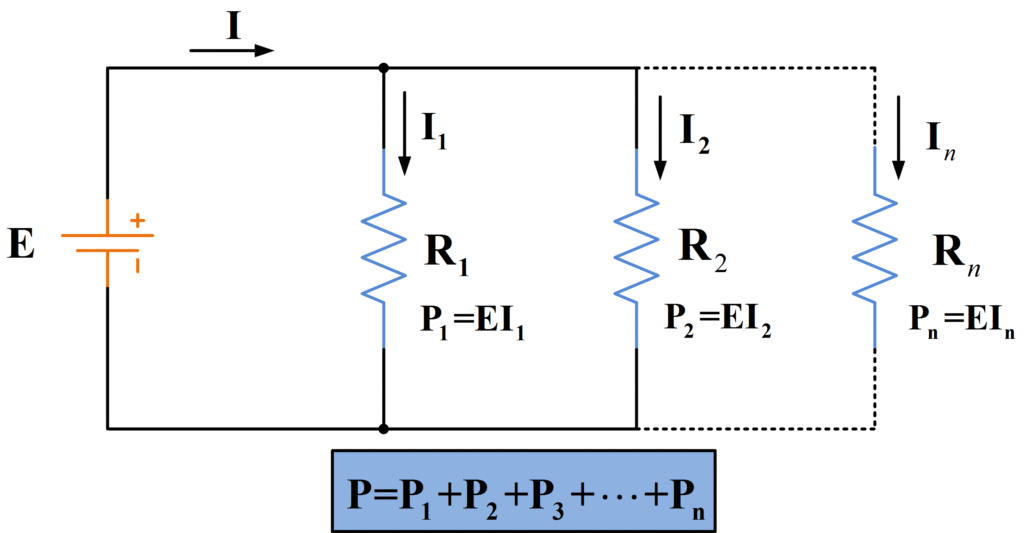Fig.6: Power Dissipation in Parallel Resistor Circuit

## Parallel Circuit Example 5

For the circuit described in figure 4 (above example 3), calculate the power dissipated in R1 and R2 and the total power supplied from the battery.

Solution

\begin{align} & {{P}_{1}}=\frac{{{E}^{2}}}{{{R}_{1}}}=\frac{{{9}^{2}}}{12}=6.75W \\ & {{P}_{2}}=\frac{{{E}^{2}}}{{{R}_{2}}}=\frac{{{9}^{2}}}{15}=5.4W \\\end{align}

Also,

$P={{P}_{1}}+{{P}_{2}}=6.75+5.4=12.15W$

## Open Circuit and Short Circuit in a Parallel Circuit

When one of the components in a parallel resistance circuit is open-circuited, as illustrated in figure 7, no current flows through that branch of the circuit. The other branch currents are not affected by such an open circuit because each of the other resistors still has full supply voltage applied its terminals.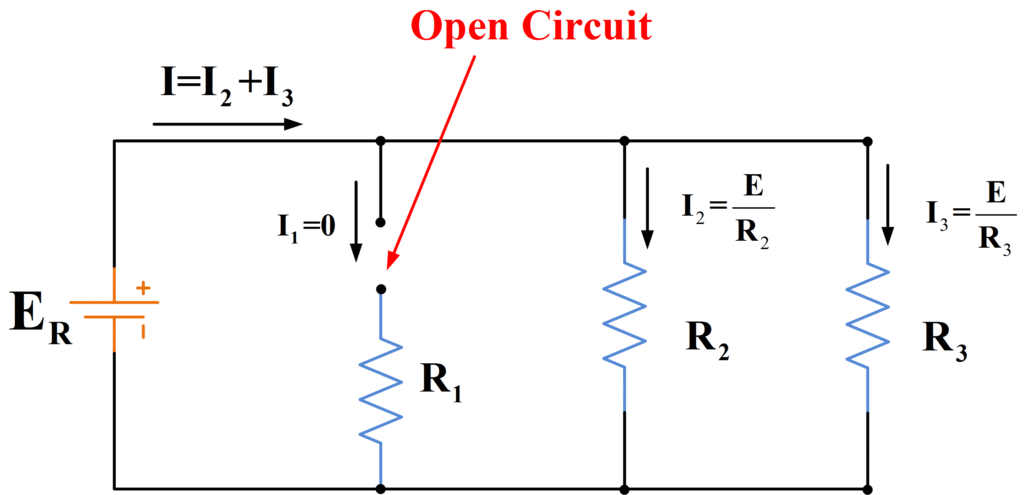Fig.7: Open-Circuited Resistor

When I1 goes to zero, the total current drawn from the battery is reduced from

$I={{I}_{1}}+{{I}_{2}}+{{I}_{3}}$

To

$I={{I}_{1}}+{{I}_{2}}$

Figure 8 shows a short-circuit across a resistor R3. This has the same effect whether it is across R1, R2, or R3, or across the voltage source terminals. In this case, the current that flows through each resistor is effectively zero. However, the battery now has a short-circuit across its terminals. Consequently, the battery short-circuit current flows:

${{I}_{sc}}=\frac{E}{{{r}_{i}}}$

Where ri is the battery internal resistance. In this situation, abnormally large current flow, and the battery could be seriously damaged.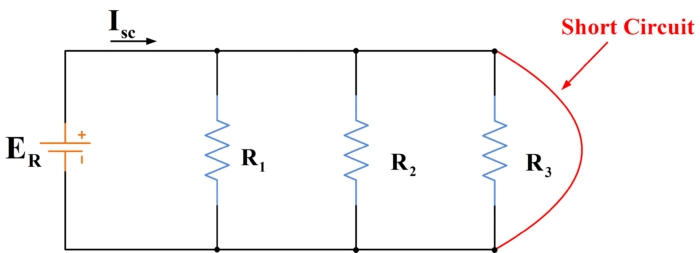Fig.8: Short-Circuit across a Resistor

Did you find apk for android? You can find new Free Android Games and apps.